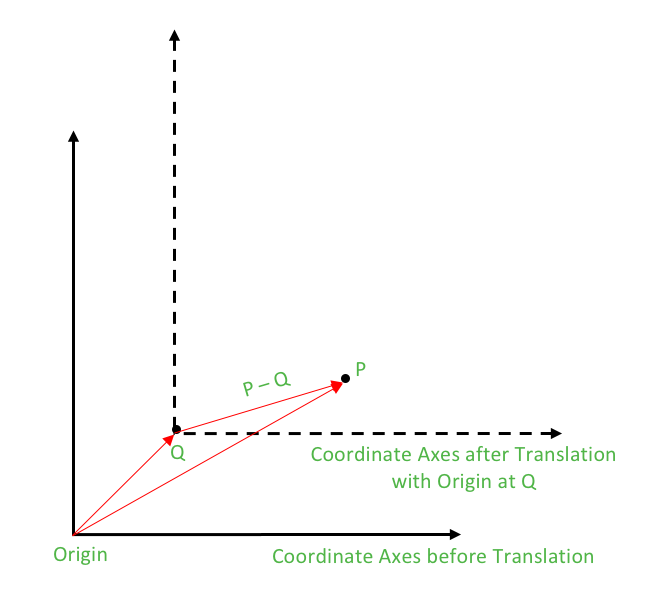GeeksforGeeks App
Open AppBrowser
Continue

# Rotation of a point about another point

We have already discussed the rotation of a point P about the origin in the Set 1 and Set 2. The rotation of point P about origin with an angle θ in the anti-clockwise direction is given as under:

`Rotation of P about origin: P * polar(1.0, θ)`

Rotation of P about point Q

Now, we have to rotate the point P not about origin but about a general point Q. This can be easily understood by the method of translation which is quite a common technique adopted in geometric analysis. What is Translation? In Euclidean geometry, translation is a geometric transformation that moves every point of a figure or a space by the same amount in a given direction. How to Perform Translation? Translation can also be interpreted as the addition of a constant vector to every point, or as shifting the origin of the coordinate system. After the translation, required computations are made and the translation is nullified by subtracting the constant vector to every point or shifting the origin back.So, for rotating P about Q, we shift the origin at Q i.e. we subtract the vector equivalent of Q from every point of the coordinate plane. Now the new point P – Q has to be rotated about the origin and then translation has to be nullified. These steps can be described as under:

1. Translation (Shifting origin at Q): Subtract Q from all points. Thus, P becomes P – Q
2. Rotation of (P – Q) about origin: (P – Q) * polar(1.0, θ)
3. Restoring back the Origin: Add Q to all the points.

Thus,

` Rotation of P about Q : (P – Q) * polar(1.0, θ) + Q`

## CPP

 `// CPP example to illustrate the rotation``// of a point about another point``#include ``#include ` `using` `namespace` `std;` `typedef` `complex<``double``> point;``#define x real()``#define y imag()` `// Constant PI for providing angles in radians``#define PI 3.1415926535897932384626` `// Function used to display X and Y coordinates of a point``void` `displayPoint(point P)``{``    ``cout << ``"("` `<< P.x << ``", "` `<< P.y << ``")"` `<< endl;``}` `//Function for Rotation of P about Q by angle theta``point rotate(point P, point Q, ``double` `theta)``{``    ``return` `(P-Q) * polar(1.0, theta) + Q;``}` `int` `main()``{``    ``// Rotate P about Q``    ``point P(4.0, 3.0);``    ``point Q(2.0, 2.0);` `    ``// Angle of rotation = 90 degrees``    ``double` `theta = PI/2;``    ` `    ``point P_rotated = rotate(P, Q, theta);``    ``cout << ``"The point P on rotating 90 degrees anti-clockwise about Q becomes:"``;``    ``cout << ``"P_rotated"``; displayPoint(P_rotated);` `    ``return` `0;``}`

## Java

 `import` `java.util.*;``import` `java.lang.*;``import` `java.io.*;``import` `java.util.stream.*;` `class` `Main``{``    ``public` `static` `void` `main (String[] args) ``throws` `java.lang.Exception``    ``{``        ``// Rotate P about Q``        ``Point P = ``new` `Point(``4.0``, ``3.0``);``        ``Point Q = ``new` `Point(``2.0``, ``2.0``);` `        ``// Angle of rotation = 90 degrees``        ``double` `theta = Math.PI / ``2``;` `        ``Point P_rotated = rotate(P, Q, theta);``        ``System.out.print(``"The point P on rotating 90 degrees anti-clockwise about Q becomes: "``);``        ``displayPoint(P_rotated);``    ``}` `    ``// Function used to display X and Y coordinates of a point``    ``static` `void` `displayPoint(Point P)``    ``{``        ``System.out.println(``"("` `+ P.x + ``", "` `+ P.y + ``")"``);``    ``}` `    ``// Function for Rotation of P about Q by angle theta``    ``static` `Point rotate(Point P, Point Q, ``double` `theta)``    ``{``        ``return` `(P.subtract(Q)).multiply(``new` `Point(Math.cos(theta), Math.sin(theta))) .add(Q);``    ``}` `    ``static` `class` `Point``    ``{``        ``double` `x;``        ``double` `y;` `        ``Point(``double` `x, ``double` `y)``        ``{``            ``this``.x = x;``            ``this``.y = y;``        ``}` `        ``Point add(Point b)``        ``{``            ``return` `new` `Point(x + b.x, y + b.y);``        ``}` `        ``Point subtract(Point b)``        ``{``            ``return` `new` `Point(x - b.x, y - b.y);``        ``}` `        ``Point multiply(Point b)``        ``{``            ``return` `new` `Point(x * b.x - y * b.y, x * b.y + y * b.x);``        ``}` `        ``Point multiply(``double` `b)``        ``{``            ``return` `new` `Point(x * b, y * b);``        ``}` `        ``Point conjugate()``        ``{``            ``return` `new` `Point(x, -y);``        ``}` `        ``double` `mod()``        ``{``            ``return` `Math.sqrt(x * x + y * y);``        ``}` `        ``double` `arg()``        ``{``            ``return` `Math.atan2(y, x);``        ``}` `        ``Point polar(``double` `r, ``double` `theta)``        ``{``            ``return` `new` `Point(r * Math.cos(theta), r * Math.sin(theta));``        ``}``    ``}``}` `//This code is contributed by Gaurav_Arora`

Output:

`The point P on rotating 90 degrees anti-clockwise about Q becomes: P_rotated(1, 4)`

Time Complexity: O(1)

Auxiliary Space: O(1)

To rotate a point about another point in a two-dimensional coordinate system, you can follow these steps:

Identify the coordinates: Let’s assume you have two points: the point you want to rotate (let’s call it P), with coordinates (Px, Py), and the point about which you want to rotate (let’s call it Q), with coordinates (Qx, Qy).

1. Translate the coordinates: To simplify the rotation process, translate the coordinate system so that point Q becomes the origin (0, 0). This can be done by subtracting the coordinates of Q from the coordinates of P. Let’s denote the translated coordinates as (Px’, Py’), which are obtained as:
Px’ = Px – Qx
Py’ = Py – Qy
2. Apply rotation: Perform the rotation of the translated point (Px’, Py’) by a desired angle θ using a rotation matrix. The rotation matrix can be defined as follows:
| cos(θ) -sin(θ) |
| sin(θ) cos(θ) |
3. Multiply the rotation matrix with the translated point as follows:
Px_rotated = Px’ * cos(θ) – Py’ * sin(θ)
Py_rotated = Px’ * sin(θ) + Py’ * cos(θ)
4. Translate back: After the rotation, translate the rotated coordinates back to the original coordinate system by adding the coordinates of Q to the rotated coordinates. The final rotated point is denoted as (Rx, Ry):
Rx = Px_rotated + Qx
Ry = Py_rotated + Qy
5. The point (Rx, Ry) represents the result of rotating point P by angle θ about point Q in the two-dimensional coordinate system.

It’s worth noting that the rotation angle θ is usually specified in radians. If you have an angle in degrees, you can convert it to radians by multiplying it by (π/180). Additionally, keep in mind that the direction of rotation (clockwise or counterclockwise) depends on the coordinate system and the sign convention used for the angles.